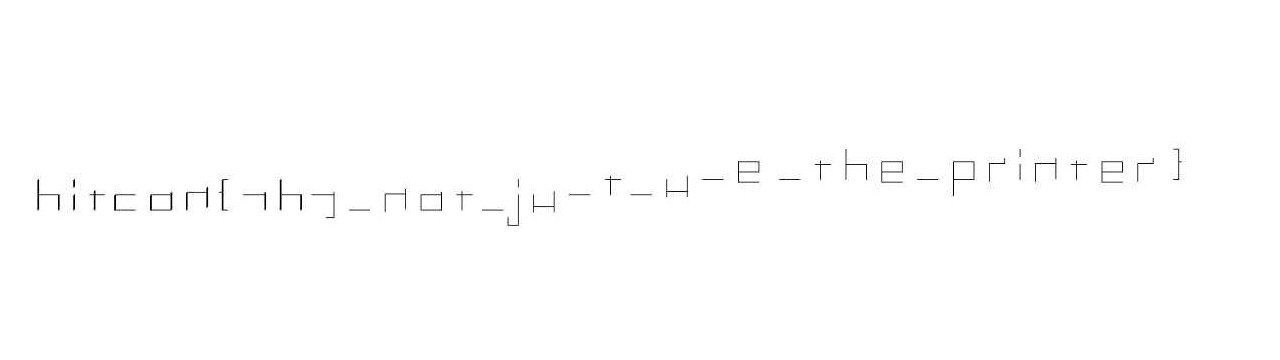# EV3 Arm - HITCON Quals 2019

tl;dr

1. Decompile the given RBF file
2. Extract the low level instructions.
3. Write a script to plot the lines.

Challenge Points: 221
Challenge Solves: 44
Solved by: f4lc0n, g4rud4,stuxn3t

## Initial Analysis

This challenge looks similar to last year’s EV3 challenge. But this time a compiled project was given instead of network logs.
So, after a couple of minutes surfing the internet about decompiling ev3 project files, I found this website which actually retrieves the low level instructions sent to the MindStorm Robot. After uploading the given rbf file to the website it listed out all the decompiled instructions in the file.

Some of the instructions looked like this

``````[[email protected] ~]\$ cat operations.txt | head

port_motor: B | rotations: 35 | speed: 15
port_motor: A | rotations: 720 | speed: 75
port_motor: C | rotations: 2.5 | speed: 70
port_motor: A | rotations: 540 | speed: -75
port_motor: B | rotations: 35 | speed: -15
port_motor: C | rotations: 2 | speed: 70
port_motor: A | rotations: 240 | speed: 75
port_motor: C | rotations: 2 | speed: -70
``````

## Analyzing the instructions

So, basically after sometime of struggling with the instructions we understood that,

1. Port Motor B is responsible for the robot’s arm. It basically determines whether the marker should be touching the paper while moving.
• Example: `port_motor: B | rotations: 35 | speed: -15`
• This instruction is responsible for the robot’s arm to move towards the ground, making the pen touch the ground.
2. Port Motor A is responsible for the horizontal movement of the robot’s arm.
• Example: `port_motor: A | rotations: 720 | speed: 75`
• With this instruction the robot’s arm moves for 720 units in the right direction.
3. Port Motor C is responsible for moving the robot forward and backward.
• Example: `port_motor: C | rotations: 2.5 | speed: 70`
• This instruction moves the robot backward for 2.5 units(The measurement here is cycles corresponding to Motor C)

## Scripting Part

Initially I thought of printing the characters to STDOUT. But that would make things more complex. So, I decided to use PIL to plot the lines.

Now, let’s write a function that will draw a line on image.

``````def drawLine(x1,y1,x2,y2):
global im
draw = ImageDraw.Draw(im)
draw.line((x1,y1, x2,y2), fill=255)
draw.line((x1+1,y1+1, x2+1,y2+1), fill=255)
draw.line((x1-1,y1-1, x2-1,y2-1), fill=255)
``````

So, now we will write a check function for drawing the lines according to the instructions.

``````def check(arg):
motor = f[arg].split('motor: ')[:1]
if motor == 'A':
direction = f[arg].split('speed: ')
length = int(f[arg].split('rotations: ').split(' | '))/10
if int(direction) > 0:
newCoord = (coords+length,coords)
else:
newCoord = (coords-length,coords)
if OnPaper is True:
drawLine(coords,coords,newCoord,newCoord)
coords = newCoord
else:
coords = newCoord
elif motor == 'B':
if int(f[arg].split('speed: ')) < 0:
OnPaper = True
else:
OnPaper = False
else:
if int(f[arg].split('speed: ')) < 0:
direction = -1
else:
direction = +1
length = 24*float((f[arg].split('rotations: ').split(' | ')))
if direction > 0:
newCoord = (coords,coords+length)
else:
newCoord = (coords,coords-length)
if OnPaper is True:
drawLine(coords,coords,newCoord,newCoord)
coords = newCoord
else:
coords = newCoord
``````

Summing up with the two functions, the final script is,

``````#!/usr/bin/env python2
from PIL import Image, ImageDraw

def check(arg):
global OnPaper, f, im, coords
motor = f[arg].split('motor: ')[:1]
if motor == 'A':
direction = f[arg].split('speed: ')
length = int(f[arg].split('rotations: ').split(' | '))/10
if int(direction) > 0:
newCoord = (coords+length,coords)
else:
newCoord = (coords-length,coords)
if OnPaper is True:
drawLine(coords,coords,newCoord,newCoord)
coords = newCoord
else:
coords = newCoord
elif motor == 'B':
if int(f[arg].split('speed: ')) < 0:
OnPaper = True
else:
OnPaper = False
else:
if int(f[arg].split('speed: ')) < 0:
direction = -1
else:
direction = +1
length = 24*float((f[arg].split('rotations: ').split(' | ')))
if direction > 0:
newCoord = (coords,coords+length)
else:
newCoord = (coords,coords-length)
if OnPaper is True:
drawLine(coords,coords,newCoord,newCoord)
coords = newCoord
else:
coords = newCoord

def drawLine(x1,y1,x2,y2):
global im
draw = ImageDraw.Draw(im)
draw.line((x1,y1, x2,y2), fill=255)
draw.line((x1+1,y1+1, x2+1,y2+1), fill=255)
draw.line((x1-1,y1-1, x2-1,y2-1), fill=255)

def main():
global f,coords, OnPaper,im
coords = (130,10)
OnPaper=False
im = Image.new('RGBA', (260, 3000), (255,255,255))
for i in range(len(f)):
check(i)
im.save('flag.png','PNG')

if __name__=='__main__':
main()
``````

## FlagAfter correcting a few characters, we get the flag
Flag: `hitcon{why_not_just_use_the_printer}`